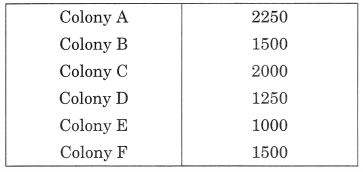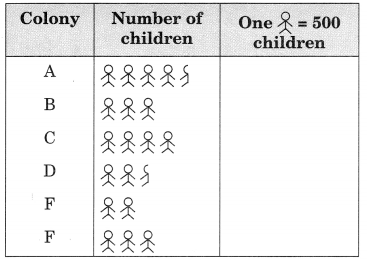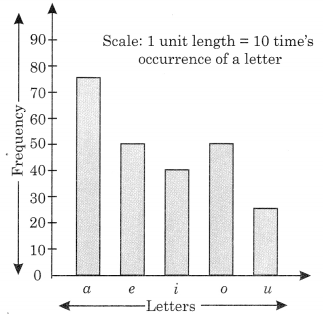# Data Handling Class 6 Extra Questions Maths Chapter 9

ncert textbook

## Data Handling Class 6 Extra Questions Maths Chapter 9

Extra Questions for Class 6 Maths Chapter 9 Data Handling

### Data Handling Class 6 Extra Questions Very Short Answer Type

Question 1.
A die was thrown 35 times and the following numbers were obtained:
5, 1, 4, 2, 3, 2, 6, 6, 1, 4, 2, 5, 4, 5, 3, 6, 1, 5
2, 6, 2, 5, 4, 1, 3, 2, 1, 4, 1, 6, 2, 6, 3, 3, 3
Prepare a frequency table for the data.
Solution:
From the given data, we have the following table.Question 2.
The result of a Mathematics test is as follows:
80, 90, 70, 80, 80, 60, 80, 70, 90, 65, 100, 60, 70, 60, 70, 85, 65, 70, 70, 85, 90, 60, 65, 80, 60
Make a frequency table for the above data and answer the following questions:
(a) What is the maximum marks obtained?
(b) How many students score less than 75 marks?
(c) How many students scored 80 marks or above?
(d) How many students appeared in the test?
Solution:
From the above information, we have the following table.(a) Maximum marks obtained by a student = 100
(b) 5 + 3 + 6 = 14 students obtained marks less than 75.
(c) 5 + 2 + 3 + 1 = 11 students scored marks 80 or above 80.
(d) Total 25 students were appeared in the test.

Question 3.
Mr. Rajan made a pictograph given below to show the number of cars washed at a car washing station during three days of a week.From the pictograph, find that:
(a) How many cars were washed on
(i) Friday
(ii) Saturday
(iii) Sunday?
(b) On which day the maximum number of cars were washed at the station?
(c) On which day the minimum number of cars were washed at the station?
(d) How many more cars were washed on Saturday than on Friday?
Solution:
(a) (i) On Friday – 4 x 5 = 20 cars
(ii) On Saturday – 9 x 5 = 45 cars
(iii) On Sunday – 7 x 5 = 35 cars.
(b) On Saturday, the maximum number of cars,
i.e., 9 x 5 = 45 were washed at the stations.
(c) On Friday, the minimum number of cars,
i.e., 4 x 5 = 20 were washed on the station.
(d) 45 – 20 = 25 more cars were washed on Saturday than on Friday.

Question 4.
Persons employed in one year(a) What is the number of persons employed in government service?
(b) How many more person were employed in government service than in private service?
(c) In which service, were the maximum number of persons employed?
Solution:
(a) Number of persons employed in government service = 10 x 3000 = 30,000
(b) 10 x 3000 – 6 x 3,000 = 30,000 – 18,000 = 12,000 persons were employed more in government service than in private service.
(c) In government service, the maximum number of persons were employed.

Question 5.
In March 2012, children for six colonies of Meerut were given pulse polio Drops. The colony wise number of children were as follows:Represent the data by pictograph.
Solution:
Pictograph:Question 6.
The given bar graph represents the frequency of a, e, i, o, and u in a piece of English writing.(a) Which letter occurred the maximum number of times?
(b) Which letter occurred 40 times?
(c) Which letter occurred less than 30 times?
(d) Write down the five letters in the decreasing order of frequencies.
Solution:
(a) a letter occurred the maximum number of times.
(b) i letter occurred 40 times.
(c) u letter occurred less than 30 times.
(d) a, e, o, i, u is the decreasing order of their frequencies.

Question 7.
The marks obtained by six students in Mathematics are given below. Represent the. data by a bar graph. Use a scale of 0.5 cm for each name on the horizontal axis and 0.5 cm for 10 marks on the vertical axis.Solution:
The required bar graph is given as below: